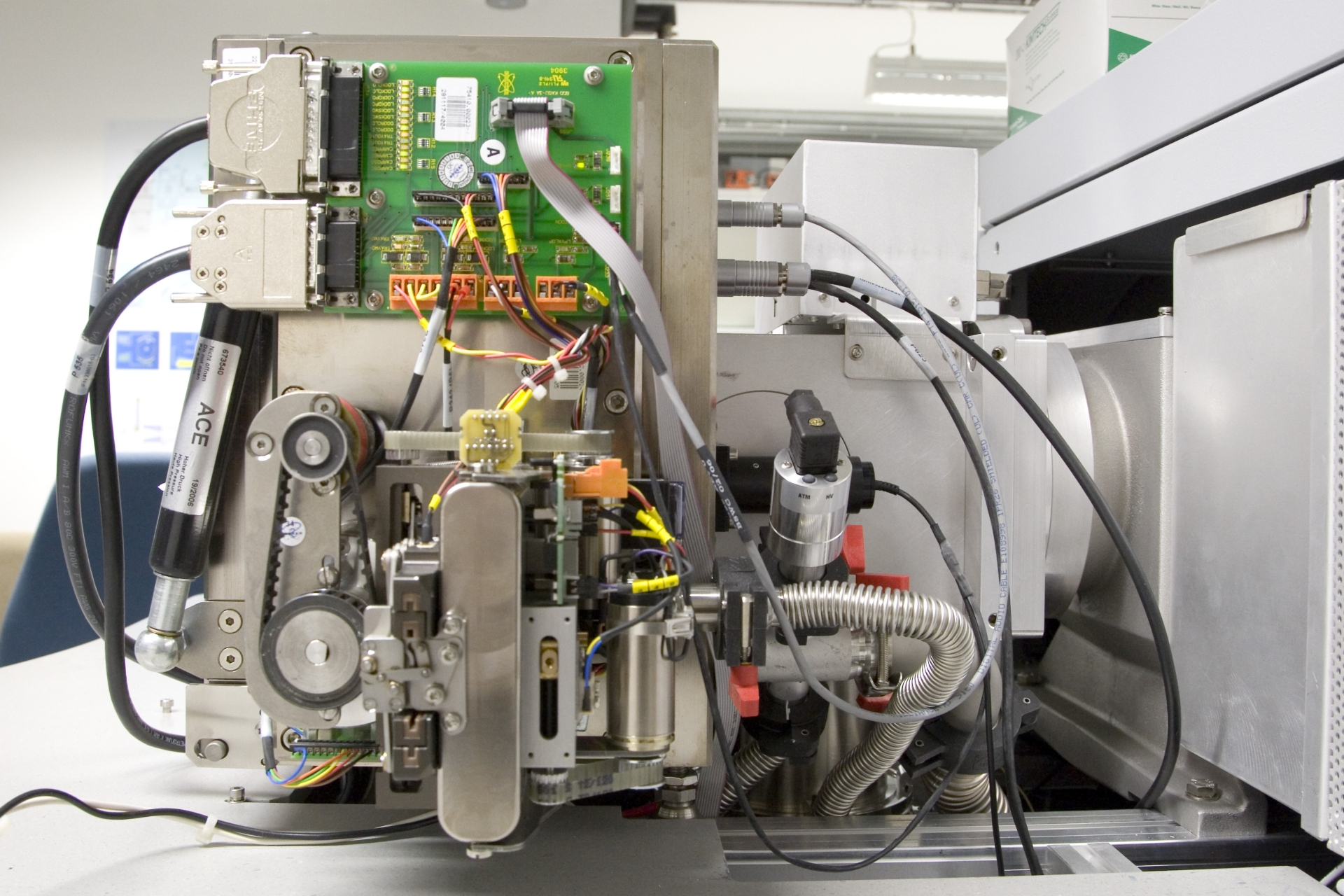# The Mass of Atoms and Molecules

We are perfectly used to the determination of the mass of a macroscopic object. Commonly, we use scales for this type of measurement. Strictly speaking, a pair of scales measures the weight of an object as the result of being subjected to the Earth’s gravitation.

Mass is a physical quantity that is independent of local gravitation. We all know the examples of astronauts on Moon where they experience just 1/6 of their weight on Earth. However, their mass is still the same independent of where they are.
The unit of mass is the kilogram (kg).

The masses of atoms and molecules are extremely small, by far beyond our imagination. The amount of 12.0 g of carbon corresponds to the amount of substance we define as one mole (1 mol). The mole is defined as a number of particles, 6.022 ´ 1023 particles to be accurate.

To calculate the mass of a single atom of carbon, we just need to divide the molar mass of 12.0 g (0,012 kg) by the number of particles per mole (Avogadro’s number). Doing so yields 1.99 ´ 10–26 kg as the mass of a carbon atom. Large molecules, in particular macromolecules are composed of many atoms. Still, even a large molecule like insulin, C254H377N65O75S6, has a mass of just 9.53 ´ 10–24 kg or 9.53 ´ 10–21 g.

To illustrate the tiny size of a molecule, one may compare the proportions of a molecule of ascorbinic acid (vitamin C, ca. 1 nm) to those of a grapefruit (ca. 10 cm) containing vitamin C. We find that ratio is about 1: 100.000.000. Now, the proportion of this grapefruit to Earth is also about 1:100.000.000.Thus, weighing is no more feasible. Even the most sensitive laboratory scales, capable of weighing down to about 1 µg (microgram, one millionth of a g or 10–6 g) are far away from measuring the mass of a molecule. So let us go down by several orders of magnitude: 1 ng (nanogram, one billionth of a g or 10–9 g), 1 pg (10–12 g), 1 fg (femtogram, 10–15 g), 1 ag (attogram, 10–18 g). And even the attogram is roughly by a factor of 1000 larger than the mass of a single molecule of insulin. Clearly, weighing isn’t anymore an option.

The so-called unified atomic mass (unit symbol u) serves to quantify atomic and molecular mass. The unified atomic mass is defined as 1/12 of the mass of one atom of the nuclide 12C. (The nuclide 12C represents the most abundant type of carbon atoms, about 99%, the others are 13C, and in traces the radioactive 14C). In analogy to our above calculation we obtain
1 u = 1.67 ´ 10–27 kg.

Provided we know the mass number of an atom, its mass can be calculated quite accurately by multiplying this mass number by 1 u. It may appear disappointing to some degree that this simple calculation does not yield the exact mass, but this may even be exploited to our advantage for formula determination based on measuring accurate mass.

So one carbon atom 12C possesses a mass of 12 u or 1.99 ´ 10–26 kg, one molecule of insulin has 5734 u or 9.53 ´ 10–24 kg. Clearly, using atomic mass units comes in more handy than using kilograms. They are nonetheless far beyond our imagination and it definitely requires some completely different approach for their accurate measurement: mass spectrometry!

A note concerning gigantic and minuscule numbers in science. Nature comprises enormous orders of magnitude. Factors of billions are common in natural sciences. Atmospheric pressure, for example, is 1000 mbar while high vacuum is 10–6 mbar, one billionth of atmospheric pressure. Due to the formula C12H22O11, a sugar molecule (saccharose) has a mass of 342.3 u or 5.72 ´ 10–25 kg; a grain of sugar of 0,05 mg thus corresponds to 1.46 ´ 10–5 mol or 88 trillion molecules. Anything outside our daily reality may appear as something very special or even unreal. Just accept these orders of magnitude as something natural.